794

Physics Properties Of Matter Level: High School

Bernoulli’s theorem

A curve ball’s path bends to the right as it flies towards home plate because it experiences an aerodynamic force to its right . One reason the ball is experiencing this aerodynamic lift force is that the ball is spinning , and that spin

exerts a torque on the ball that causes its angular momentum to shift towards the left .

causes the air to flow more rapidly around the right side of the ball than around the left side of the ball .

Exerts a torque on the ball that causes its angular momentum to shift towards the right .

Causes the air to flow more rapidly around the left side of the ball than around the right side of the ball .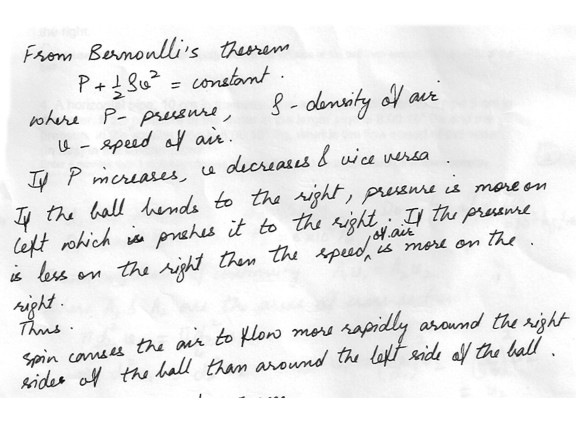793

Physics Properties Of Matter Level: High School

Bernoulli’s theorem

An airplane wing with an average cross a sectional area of 10m^2 experiences a lift force of 5000 N . What is the difference in air pressure (in Pa) , on the average , between the bottom and the top of the wing ?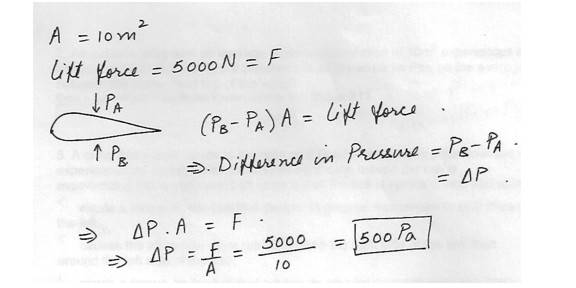792

Physics Properties Of Matter Level: High School

Gas

An ideal gas is held in a container at constant volume . Initially, its temperature is 10.0 degrees Celsius and its pressure is 2.50 atm .

What is the pressure of the gas if it is cooled to -20 degrees Celsius ?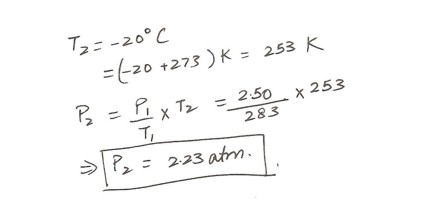791

Physics Properties Of Matter Level: High School

Gas

An ideal gas is held in a container at constant volume . Initially , its temperature is 10.0 degrees Celsius and its pressure is 2.50 atm . What is its pressure when its temperature is 80.0 degrees Celsius ?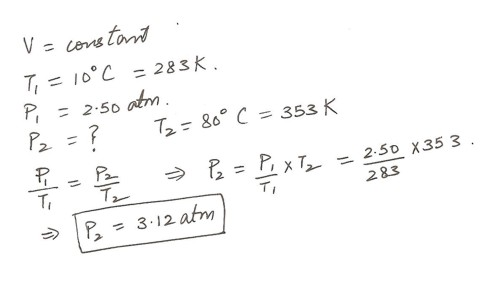783

Physics Properties Of Matter Level: High School

Solids

Suppose the ends of the rail are rigidly clamped at 0degreeC to prevent expansion . Calculate the thermal stress in the rail if its temperature is raised to 37degreeC . The Young modulus for steel is 20 x 10^10 N/m^2 . Answer in units of m .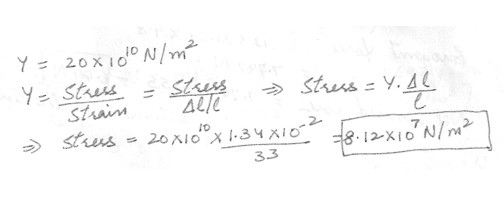777

Physics Properties Of Matter Level: High School

Solids

A steel railroad track has a length of 33 m when the temperature is 0 degreeC .

What is the increase in the length of the rail on a hot day when the temperature is 37 degree C ? The linear expansion coefficient of steel is 11 x 10^-6 (degree C)^-1 . Answer in units of m .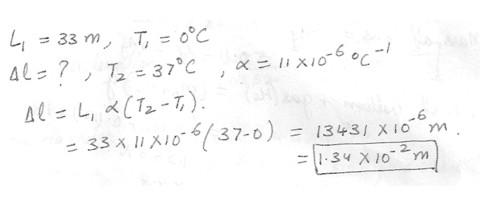776

Physics Properties Of Matter Level: High School

Solids

A bridge is made with segments of concrete 94 m long (at the original temperature ) . If the linear expansion coefficient is 1.2 x 10^-5 (degree C)^-1 . how much spacing is needed to allow for expansion for an increase in temperature of 49 degreeF ? Answer in units of cm .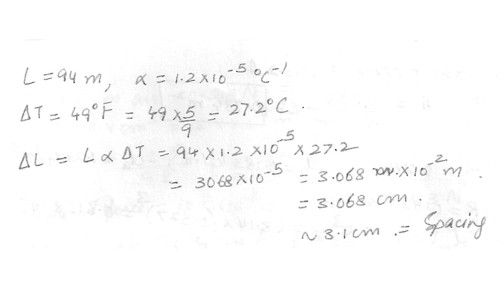775

Physics Properties Of Matter Level: High School

Solids

A building made with a steel structure is 641 m high on a winter day when the temperature is 0degree F . How much taller is the building when the temperature is T = 99 degree F ? (The linear expansion coefficient of steel is infinite = 1.1 x 10^-5 (degreeC) -1 Answer in units of cm .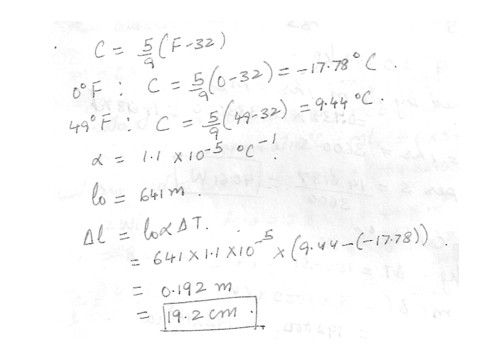774

Physics Properties Of Matter Level: High School

Solid

An empty rubber balloon has a mass of 0.0161 kg . The balloon is filled with helium at a density of 0.183 kg/m^3 . At this density the balloon has a radius of 0.527 m .

The acceleration of gravity is 9.8 m/s^2. and the density of air is 1.29 kg/m^3. If the filled balloon is fastened to a vertical line, What is the tension in the line ? Answer in units of N .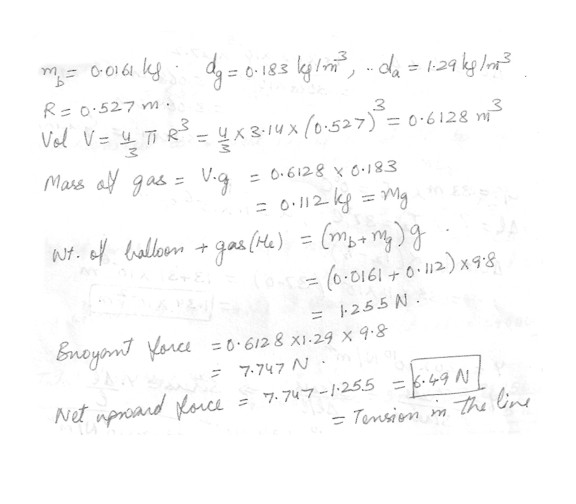773

Physics Properties Of Matter Level: High School

A Styrofoam slab has a thickness of 13.4 cm and a density of 304 kg/m^3 . What is the area of the slab if it floats just awash (top of slab is even with the water surface) in fresh water when a 79 kg swimmer is aboard ? The density of water is 1000 kg/m^3 . Answer in units of m^2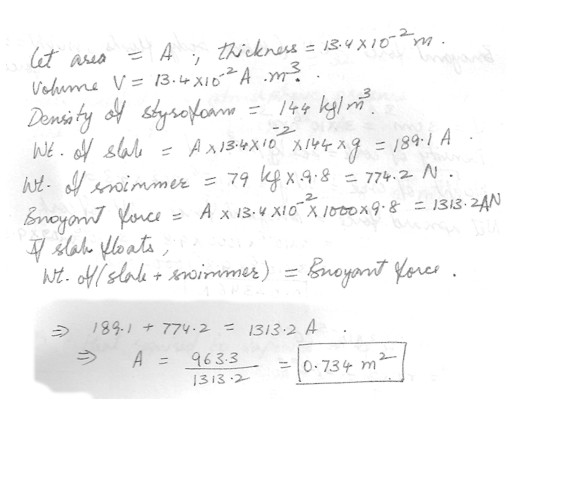772

Physics Properties Of Matter Level: High School

A Ping-Pong ball has a diameter of 4.6 cm and average density of 0.0647 g/cm^3 . What force would be required to hold it completely submerged under water ? Answer in units of N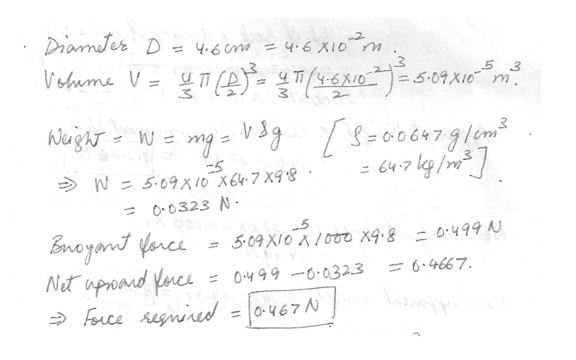771

Physics Properties Of Matter Level: High School

A small metal sphere weighs 0.345 N in air and has a volume of 13.4 cm^3 .

The acceleration of gravity is 9.8 m/s^2 . What is the acceleration of the sphere as it falls through water ? Answer in units of m/s^2 .770

Physics Properties Of Matter Level: High School

A cork is held at the bottom of a bucket of water by a piece of string . The actual depth of the cork is 0.813537 in below the surface of the water . If the density of the cork id 202 kg/m^3 and the volume of the cork is 3 cm^3 , then what is the tension in the string ? g = 9.8 m/s^2 . Assume the density of water is 1000 kg/m^3 .

1. 0.0117306 N

2. 0.0234612 N

3. 2.34612 N

4. 0.234612 N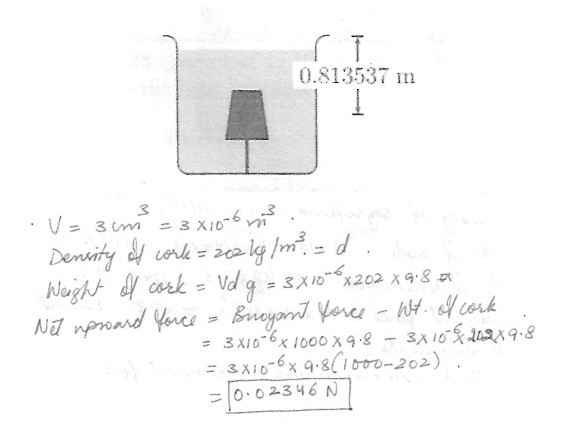769

Physics Properties Of Matter Level: High School

Archimedes Principle

A floating ball normally weighs 6N . The acceleration of gravity is 9.8 m/s^2 .

What is the size of the buoyant force that acts on it ?

Bnoyant force = 6 N

( When body floats , weight = bnoyant force )

768

Physics Properties Of Matter Level: High School

Archimedes Principle

If it is submerged in a liquid with a density exactly 2 times that of water , what will be its new apparent weight ? Answer in units of N .
New bnoyant force = 0.00278 x 2 x 1000 x 9.8 = 54.49 N

New apparent weight = 140 - 54.49 = 85.51 N

Displaying 496-510 of 522 results.